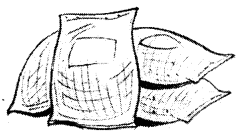AP State Syllabus AP Board 7th Class Maths Solutions Chapter 1 Integers Ex 4 Textbook Questions and Answers.

##Question 1.
Fill in the blanks.
(i) ( – 100) × ( -6) =
(ii) ( – 3) × …………. = 3
(iii) 100 × ( – 6) = ………….
(iv) ( – 20) × (- 10) = ………….
(v) 15 × (-3) = ………….
Solution:
(i) ( – 100) × ( -6) =
(ii) ( – 3) × …………. = 3
(iii) 100 × ( – 6) = ………….
(iv) ( – 20) × (- 10) = ………….
(v) 15 × (-3) = ………….

Question 2.
Find each of the following products.
(i) 3 × ( – 1)
(ii) ( – 1) × 225
(iii) ( – 2 ) × ( – 30)
(iv) ( – 316) × ( – 1)
(v) (-15) × 0 × (-18)
(vi) (-12) × (-11) × (10)
(vii) 9 × ( – 3) × ( – 6)
(viii) ( – 18) × ( – 5) × ( – 4)
(ix) ( – 1) × ( – 2) × ( – 3) × 4
(x) ( – 3) × ( – 6) × ( – 2) × ( – 1)
Solution:
(i) 3 × ( – 1) = -3
(ii) ( – 1) × 225 = -225
(iii) ( – 2 ) × ( – 30) = 630
(iv) ( – 316) × ( – 1) = 316
(v) (-15) × 0 × (-18) = 0
(vi) (-12) × (-11) × (10) = 1320
(vii) 9 × ( – 3) × ( – 6) = 162
(viii) ( – 18) × ( – 5) × ( – 4) = -360
(ix) ( – 1) × ( – 2) × ( – 3) × 4 = -24
(x) ( – 3) × ( – 6) × ( – 2) × ( – 1) = 36Question 3.
A certain freezing process requires that room temperature be lowered from 40°C at the rate of 5°C every hour. What will be the room temperature 10 hours after the process begins?
Solution:
At present, the room temperature 40°C
The total decrease in temperature after 10 hours at the rate of 5°C a hour = 10 × 5 = 50°
∴ Temperature after 10 hours = 40° – 50° = – 10°C.

Question 4.
In a class test containing 10 questions, ‘3’ marks are awarded for every correct answer and ( – 1) mark is for every incorrect answer and ‘0’ for questions not attempted.
(i) Gopi gets 5 correct and 5 incorrect answers. What is his score?
(ii) Reshma gets 7 correct answers and 3 incorrect answers. What is her score?
(iii) Rashmi gets 3 correct and 4 incorrect answers out of seven questions she attempts. What is her score?
Solution:
i) GopI’sscoe =5 × 3 + 5( – 1)= 15 + ( – 5) = 10
ii) Reshmasscore = 7 × 3 + 3 × ( – 1) = 21 +( – 3) = 18
iii) Rashmis score = 3 × 3 + 4( – 1) = 9 + ( – 4) = 5Question 5.
A merchant on selling rice earns a profit of ₹ 10 per bag of basmati rice sold and a loss of ₹ 5 per bag of 1 non-basmati rice.(i) He sells 3,000 bags of basmati rice and 5,000 bags ofnon-basmati rice in a month. What is his profit or loss in a month?
(ii) What is the number of basinati rice bags he must sell to have neither profit nor loss, if the number of bags of non-basmati rice sold is 6,400.
Solution:
i) Profit on 3,000 bags of basmati rice = 3,000 × 10 = ₹ 30,000
Loss on 5,000 bags of non-basmati rice = 5,000 × 5 = ₹ 25,000
Net profit or loss = 30,000 – 25,000 = ₹ 5000 profit
Another Method : Net profit or loss = 3,000 × 10 + 5,000(-5)
= 30,000 – 25,000 = ₹ 5,000

ii) As there is no loss or gain
Profit on basmati rice= Loss on non-basmati rice
But loss on nonbasrnati rice = 6,400 × 5 = ₹ 32,000
∴ Number of bags of basmati rice sold = $$\frac{32,000}{10}$$ = 3200Question 6.
Replace the blank with an integer to make it a true statement.
(i) (- 3) × ______________ = 27
(ii) 5 × ______________ = – 35
(iii) _______ × ( – 8) = – 56
(iv) _______ × ( – 12) = 132
Solution:
(i) (- 3) × -9 = 27
(ii) 5 × -7 = – 35
(iii) 7 × ( – 8) = – 56
(iv) 11 × ( – 12) = 132